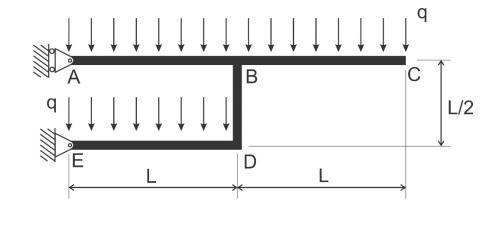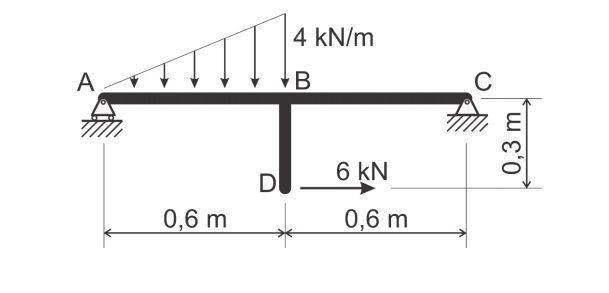# Internal Forces Diagram: how to do this one?

I'm wondering how should I do analysis on those problems below:
Figure 1:Figure 2:I mean, my professor, while doing an example very similar to Figure 2, he didn't do an imaginary cut in the region BD to find internal forces there, and the internal forces diagram that he drew only contained internal forces in sections AB and BC. I'm confused by it. When drawing internal forces diagrams, how should I handle those kinds of problems?

•berkeman

PhanthomJay
Homework Helper
Gold Member
After finding reactions, you should determine the internal forces and moments in all members at the joints. Then you can draw shear and moment diagrams to determine the internal forces and moments in between the joints.

After finding reactions, you should determine the internal forces and moments in all members at the joints. Then you can draw shear and moment diagrams to determine the internal forces and moments in between the joints.
Okay, so let's consider figure 2. When I draw shear and moment diagrams, I plot internal forces in function of x - where x is the distance related to the origin, let's say, point A. But the structure has joints in other directions - y direction, for instance. So, in this case, I have to draw six diagrams? Three for normal, shear and moment internal forces in the x structure, and another 3 for the joint in the y direction?
I'm confused.

PhanthomJay